1. Part A: Each correct answer is worth 3 points

A lady bug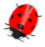will sit on a flower that has five petals and three leaves. On which of the following flowers will the ladybug sit?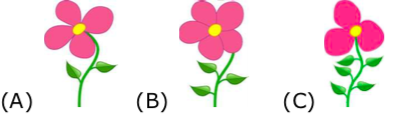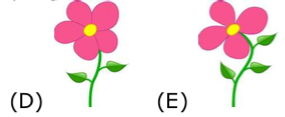2. A square was made of 25 small squares, but some of these small squares were lost. How many small squares were lost?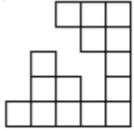3. The kangaroo is inside how many circles?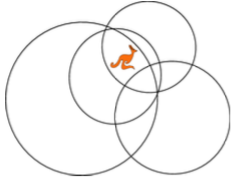4. Seven sticks lie on top of each other. Stick number 2 is at the bottom. Stick number 6 is at the top. Which stick is in the middle?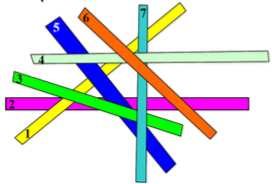5. Alan is five years old. His sister Bethany is seven years older than him. What is the sum of their ages?

6. What numbers of dots are hidden behind the cat and the dog in the equations?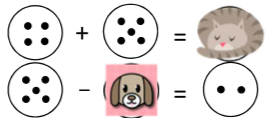7. Part B: Each correct answer is worth 4 points

The first two scales in the picture are balanced. How many ducks are needed on the right side of the third scale, to balance with the crocodile?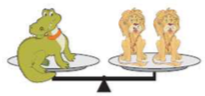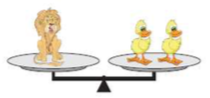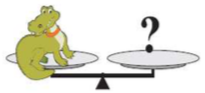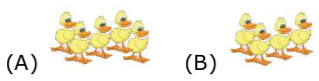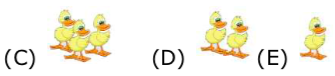8. Which of the shapes below should be placed on top of the shape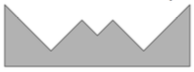to make a rectangle?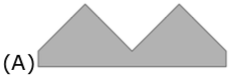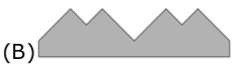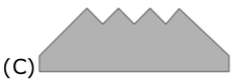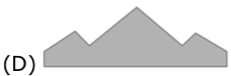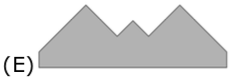9. How many numbers between 10 and 31 (including 31) can be written using only the digits 1, 2 and 3? You can repeat digits.

10. My rabbit eats only cabbage and carrots. Last week he ate either 10 carrots or 2 heads of cabbage each day. If he ate a total of 6 heads of cabbage last week, how many carrots did he eat?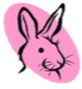11. Mary has 13 flowers, five of which are roses. The rest are tulips. Six of the flowers are white, and the remaining flowers are red. At least how many tulips are red?

12. Six little elephants, A, B, C, D, E, F, are lining up to buy tickets. F is after A and before D and he is also between B and C; B is the first in the line; and E is the last in the line. Which elephant is F?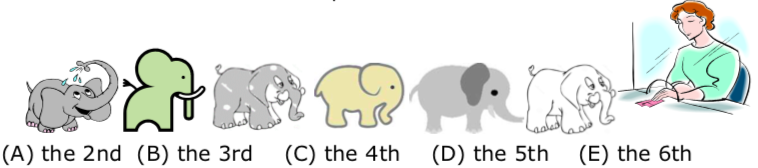13. Part C: Each correct answer is worth 5 points

A square was cut into four parts as shown in the picture.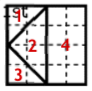Which of the following shapes cannot be made with these 4 parts?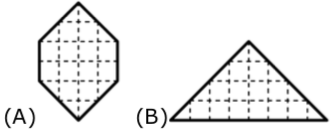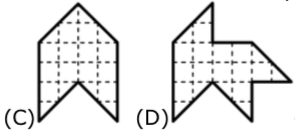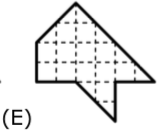14. Place the digits 2, 3, 4, and 5 in the squares so that the sum is as large as possible. What is this sum?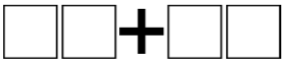15. Fedya has 4 red cubes, 3 blue cubes, 2 green cubes, and 1 yellow cube. He is building a tower (see the picture) in such a way that no two cubes touching each other have the same colour. What is the colour of the cube with the question mark?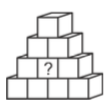16. Gear A is about to make one complete turn in the direction shown in Figure 1.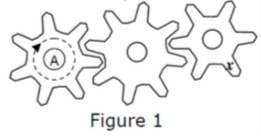The piece “x” moved to one of the positions a, b, c, d, e shown in Figure 2.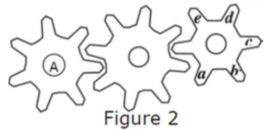To which position did “x” move?

17. How many triangles are there in the picture?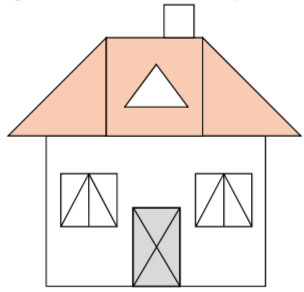18. John wrote the numbers 1, 2, 3, 4, 5, 6, 7, 8, 9 and 10 on a whiteboard. John then erased some of the numbers and added up the remaining ones. He got a sum of 24. At most how many numbers were left on the whiteboard?